Engineering Mechanics - Center of Gravity and Centroid

Why Engineering Mechanics Center of Gravity and Centroid?

In this section you can learn and practice Engineering Mechanics Questions based on "Center of Gravity and Centroid" and improve your skills in order to face the interview, competitive examination and various entrance test (CAT, GATE, GRE, MAT, Bank Exam, Railway Exam etc.) with full confidence.

Where can I get Engineering Mechanics Center of Gravity and Centroid questions and answers with explanation?

IndiaBIX provides you lots of fully solved Engineering Mechanics (Center of Gravity and Centroid) questions and answers with Explanation. Solved examples with detailed answer description, explanation are given and it would be easy to understand. All students, freshers can download Engineering Mechanics Center of Gravity and Centroid quiz questions with answers as PDF files and eBooks.

Where can I get Engineering Mechanics Center of Gravity and Centroid Interview Questions and Answers (objective type, multiple choice)?

Here you can find objective type Engineering Mechanics Center of Gravity and Centroid questions and answers for interview and entrance examination. Multiple choice and true or false type questions are also provided.

How to solve Engineering Mechanics Center of Gravity and Centroid problems?

You can easily solve all kind of Engineering Mechanics questions based on Center of Gravity and Centroid by practicing the objective type exercises given below, also get shortcut methods to solve Engineering Mechanics Center of Gravity and Centroid problems.

Exercise :: Center of Gravity and Centroid - General Questions

• Center of Gravity and Centroid - General Questions
1.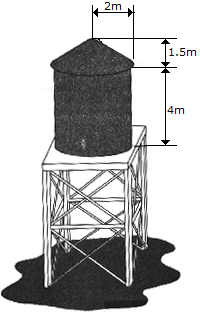Determine the approximate amount of paint needed to cover the surface of the water storage tank. Assume that a liter of paint covers 2.5 m2. Also, what is the total inside volume of the tank.

 A. 27.6 liters of paint, V = 52.6 m3 B. 20.1 liters of paint, V = 50.3 m3 C. 26.4 liters of paint, V = 56.5 m3 D. 25.1 liters of paint, V = 55.0 m3

Explanation:

No answer description available for this question. Let us discuss.

2.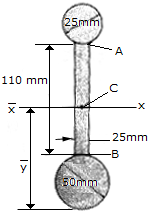Determine the distance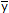to the centroid axis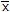of the beam's cross-sectional area. Neglect the size of the corner welds at A and B for the calculation.

 A.= 75.2 mm B.= 97.5 mm C.= 85.9 mm D.= 102.5 mm

Explanation:

No answer description available for this question. Let us discuss.

3.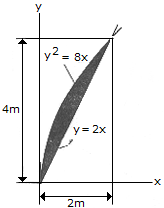Locate the centroid of the shaded area.

 A.= 0.667 m,= 2.40 m B.= 0.500 m,= 2.80 m C.= 0.800 m,= 2.00 m D.= 0.600 m,= 2.60 m

Explanation:

No answer description available for this question. Let us discuss.

4.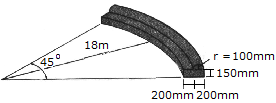Determine the volume of concrete needed to construct the circular curb.

 A. V = 1.083 m3 B. V = 1.309 m3 C. V = 1.756 m3 D. V = 8.67 m3

Explanation:

No answer description available for this question. Let us discuss.

5.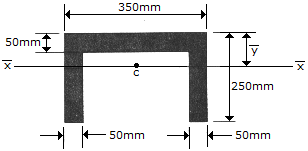Determine the distanceto the centroidal axisof the beam's cross-sectional area.

 A.= 112.3 mm B.= 125.0 mm C.= 100.0 mm D.= 91.7 mm